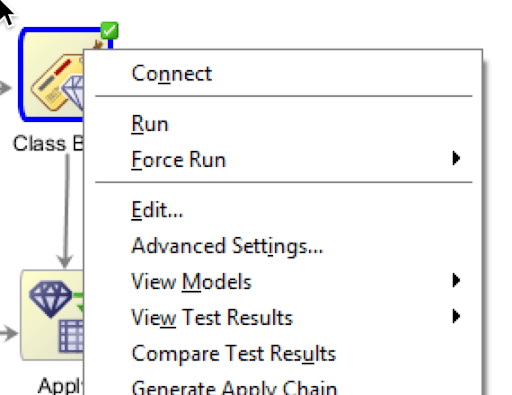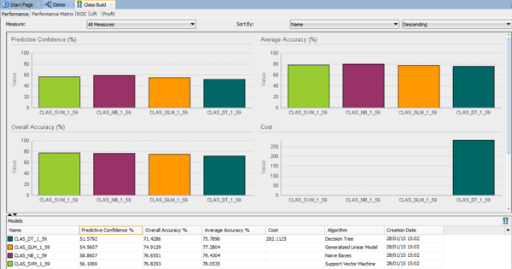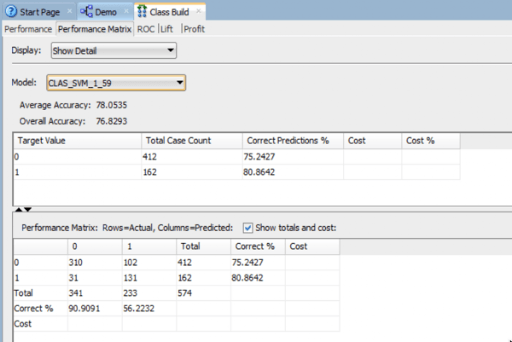### Evaluating Classification Models in ODM (Part 2)

Posted on

In a previous blog post I talked about and showed some of the typical statistical methods to evaluate the classification models that you develop. Click to see this (first) blog post.

In this blog post I want to show you how you can go about evaluating your classification models that you develop using Oracle Data Miner (part of SQL Developer).

What I’m not going to show you here is how to develop classification models using Oracle Data Mining 😦 I’ve had several blog posts over the years on this topics. So you can go and search of those posts or alternately this topic is cover in a lot more detail in my Oracle Data Miner book 🙂

After you have developed your ODM models in Oracle Data Miner you have 2 levels of details available to you. The first of these is the Compare Test Results. You can find this by right clicking on the Classification node of your ODM Workflow, as showing below.When you select the Compare Test Results a new (worksheet) tab will open. This will display summary statistics and graphics for the summary statistics for each Oracle Data Ming model created. In the following image an ODM model was created for each In-Database Classification algorithm in the Oracle Database.Here we get to see 2 of the statistical measures that I talked about in my previous blog post, the (average) Accuracy and the Overall Accuracy. We can look at and examine this in a bit more detail in a minute. A new measure that I haven’t mentioned before is the Predictive Confidence.

The Predictive Confidence measure provides an estimate of the overall goodness of the model. Predictive Confidence is a number between 0 and 1. Data Miner displays Predictive Confidence as a percent.

• If Predictive Confidence=0, then it indicates that the predictions of the model are no better than the predictions made by using the naive model.
• If Predictive Confidence=1, then it indicates that the predictions are perfect.
• If Predictive Confidence=0.5, then it indicates that the model has cut the error of a naive model by 50%./li>

So the higher the value for Predictive Confidence the better the model. Particularly when it is higher than 50%.

After evaluation these summary statistical measures you will want to drill down on these to see the lower level statistical measures, for example you will want to see the confusion matrix and the corresponding statistical measures. To view the confusion matrix all you need to do is to click on the Performance Matrix tab. Before you can really start evaluating the models you will need to click on the Display drop down and select ‘Show Detail’ from the drop down list. Another thing you will need to do is to click/check the ‘Show totals and codes’ check box on the lower part of the screen. This will give you some of the statistical measures that I outlined in my previous blog post.When you examine the statistical measures displayed on the screen you will notice that some of the statistical measures I outlined in my previous blog post are missing. Some of these missing measures are ones that you will want to consider and use as part of your evaluation of you ODM models.

So what how do you find out what these missing statistical measures are? Well ODM does not display these so the only real option open to you is to go and calculate them yourself 😦 This is not ideal but these are relatively easy to calculate and you can do this on a piece of paper or you can open your spreadsheet software and let it calculate them for you (once you have defined to formula for each). Here is an example of the completed/extended confusion matrix based on the results from the CLAS_SVM_1_59 model shown in the above image.In my next blog post I will look at how you can evaluate a classification model that was developed using the in-database Oracle Data Mining algorithms (Oracle Data Miner GUI was not used). The evaluation criteria that I will show will be based on the statistical methods that I highlighted in my first blog post on this topic.